# How to calculate formulas in a Word document

Word
In some Word documents you need to use formulas, such as summing, subtracting, multiplying, or dividing certain values:In this example was used a simple formula to calculate a discount rate:To create a formula like the one above, it is necessary to make two steps:

1.   Create a bookmark (variable) for every parameter.

2.   Create a formula.

To perform these steps, do the following:

1.   Create a field with the volume of a parameter:

1.1.   Position the cursor in the document.

It doesn't important, where: where this variable should be shown in the first time or anywhere in the text (see both examples below).

1.2.   On the Insert tab, in the Text group, select the Quick Parts drop-down list and the click on Field...: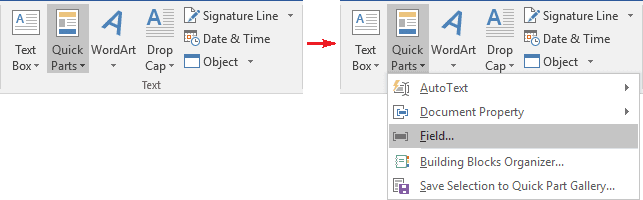1.3.   In the Field dialog box:

1.3.1.   In the Field names list, select the command Set and click the Field Codes button: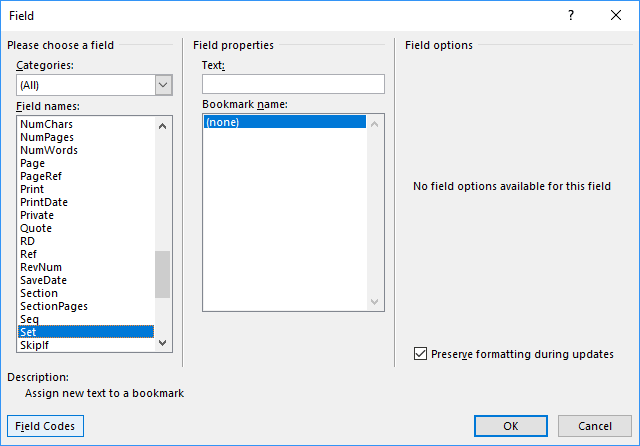1.3.2.   In the Field codes textbox, after proposed SET type the name of this bookmark (variable) and the value.

For example: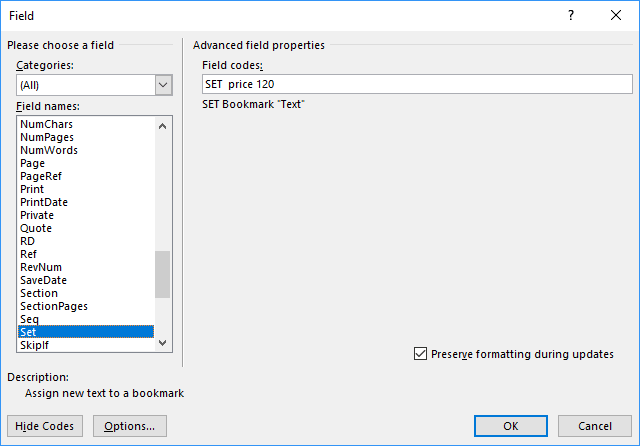1.3.3.   Click OK to insert the current field in you document: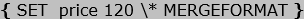Note: Instead of making steps 1.1-1.3, you can press Ctrl+F9 to insert a field in your document and type:

SET <name> <value>

To create the bookmark (variable) as you type, just update a field: right-click on the field and choose Update field in the popup menu: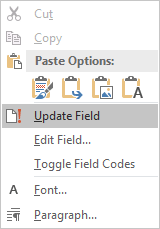2.   Repeat the step 1 for every bookmark (variable) that you want to define.

In this example, the discount with volume 5.

3.   To insert the bookmark (variable) in the text, do the following:

3.1.   Position the cursor where you want to insert the value of some bookmark (variable).

3.2.   On the Insert tab, in the Text group, select the Quick Parts drop-down list and the click on Field....

3.3.   In the Field dialog box, click the Formula... button: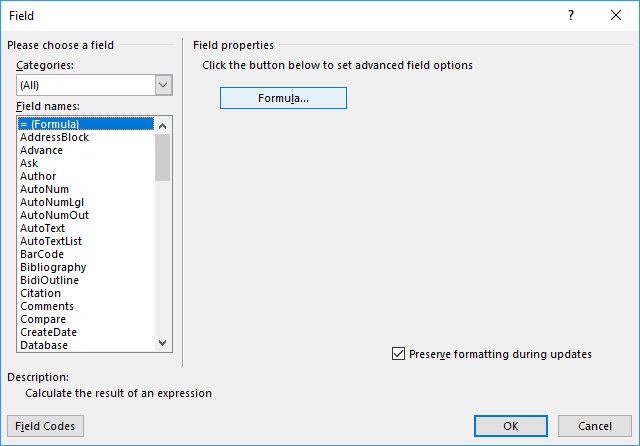3.4.   In the Formula dialog box, in the Paste bookmarks drop-down list, if you made all correctly on the previous steps, you will see all variables that you have created: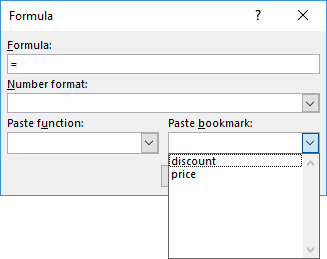Choose one and click OK. For example, price: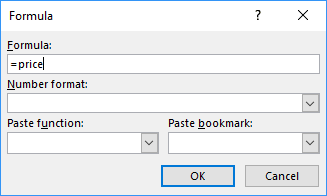Note: Instead of making steps 3.1 – 3.4, if you can press Ctrl+F9 to insert a field in you document and type:

= <bookmark name>

4.   To insert a formula, do the following:

4.1.   Position the cursor where you want to insert the formula.

4.2.   On the Insert tab, in the Text group, select the Quick Parts drop-down list and the click on Field....

4.3.   In the Field dialog box, click the Formula... button.

4.4.   In the Formula dialog box type the formula:

The first variant where each of the variables defined before their values in the text: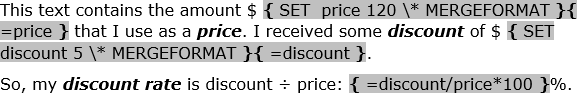The second variant where the variables defined before the text: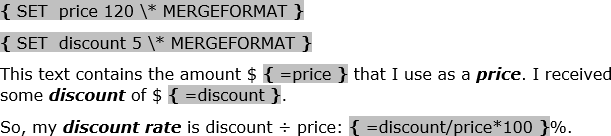See Functions and formulas in a Word document for more details.

Note: The field { SET *** } doesn't shown in the document by default. So, this field can be easily removed by mistake with any other text or paragraph. To avoid missing this field, we recommend use a field { Quote *** } to keep the definition and the first using of the parameter: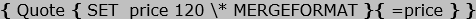See also this tip in French: Comment calculer des formules dans un document Word.